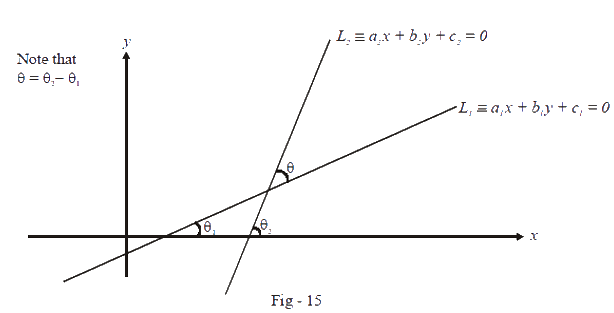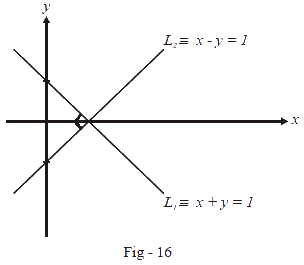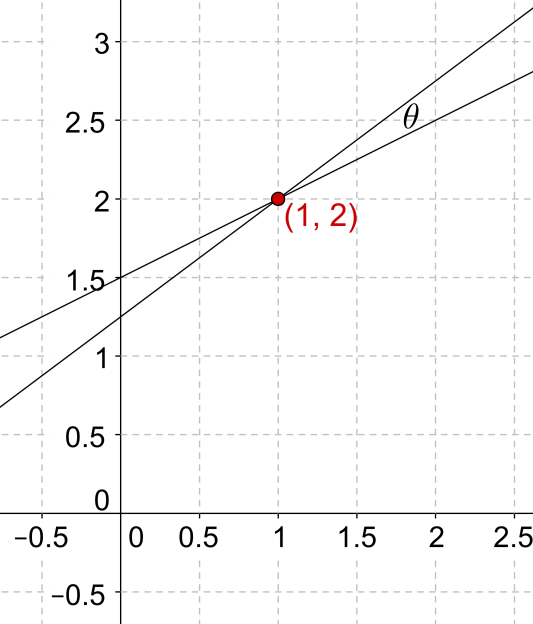In the verge of coronavirus pandemic, we are providing FREE access to our entire Online Curriculum to ensure Learning Doesn't STOP!

# Intersection of Two Lines

Go back to  'Coordinate-Geometry'

We are given two lines $${L_1}$$ and $${L_2}$$ , and we are required to find the point of intersection (if they are non-parallel) and the angle at which they are inclined to one another, i.e., the angle of intersection. Evaluating the point of intersection is a simple matter of solving two simultaneous linear equations. Let the equations of the two lines be (written in the general form):

$\begin{array}{l}{a_1}x + {b_1}y + {c_1} = 0\\{a_2}x + {b_2}y + {c_2} = 0\end{array}$

Now, let the point of intersection be $$\left( {{x_0},{y_0}} \right)$$. Thus,

$\begin{array}{l}{a_1}{x_0} + {b_1}{y_0} + {c_1} = 0\\{a_2}{x_0} + {b_2}{y_0} + {c_2} = 0\end{array}$

This system can be solved using the Cramer’s rule to get

$\frac{{{x_0}}}{{{b_1}{c_2} - {b_2}{c_1}}} = \frac{{ - {y_0}}}{{{a_1}{c_2} - {a_2}{c_1}}} = \frac{1}{{{a_1}{b_2} - {a_2}{b_1}}}$

From this relation we obtain the point of intersection $$\left( {{x_0},{y_0}} \right)$$ as

$\left( {{x_0},{y_0}} \right) = \left( {\frac{{{b_1}{c_2} - {b_2}{c_1}}}{{{a_1}{b_2} - {a_2}{b_1}}},\frac{{{c_1}{a_2} - {c_2}{a_1}}}{{{a_1}{b_2} - {a_2}{b_1}}}} \right)$

To obtain the angle of intersection between these two lines, consider the figure below:The equations of the two lines in slope-intercept form are:

\begin{align}&y = \left( { - \frac{{{a_1}}}{{{b_1}}}} \right)x + \left( {\frac{{{c_1}}}{{{b_1}}}} \right) = {m_1}x + {C_1}\\&y = \left( { - \frac{{{a_2}}}{{{b_2}}}} \right)x + \left( {\frac{{{c_2}}}{{{b_2}}}} \right) = {m_2}x + {C_2}\end{align}

Note in the figure above that $$\theta = {\theta _2} - {\theta _1}$$, and thus

\begin{align}&\tan \theta = \tan \left( {{\theta _2} - {\theta _1}} \right) = \frac{{\tan {\theta _2} - \tan {\theta _1}}}{{1 + \tan {\theta _1}\tan {\theta _2}}}\\&\qquad\qquad\qquad\qquad\;\;= \frac{{{m_2} - {m_1}}}{{1 + {m_1}{m_2}}}\end{align}

Conventionally, we would be interested only in the acute angle between the two lines and thus we have to have $$\tan \theta$$ as a positive quantity. So in the expression  above, if the expression $$\frac{{{m_2} - {m_1}}}{{1 + {m_1}{m_2}}}$$ turns out to be negative, this would be the tangent of the obtuse angle between the two lines; thus, to get the acute angle between the two lines, we use the magnitude of this expression.

Therefore, the acute angle $$\theta$$   between the two lines is

$\theta = {\tan ^{ - 1}}\left| {\frac{{{m_2} - {m_1}}}{{1 + {m_1}{m_2}}}} \right|$

From this relation, we can easily deduce the conditions on $${m_1}$$ and $${m_2}$$ such that the two lines $${L_1}$$ and $${L_2}$$ are parallel or perpendicular.

• If the lines are parallel, $$\theta = 0$$ , so that $${m_1} = {m_2}$$ , which is intuitively obvious since parallel lines must have the same slope.

• For the two lines to be perpendicular, $$\theta = \frac{\pi }{2}$$, so that $$\cot \theta = 0$$; this can happen if $$1 + {m_1}{m_2} = 0$$ or $${m_1}{m_2} = - 1$$ .

If the lines $${L_1}$$ and $${L_2}$$ are given in the general form given in the general form $$ax + by + c = 0$$, the slope of this line is $$m = - \frac{a}{b}$$ . Thus, the condition for $${L_1}$$ and $${L_2}$$ to be parallel is:

${m_1} = {m_2}\,\,\, \Rightarrow \,\,\, - \frac{{{a_1}}}{{{b_1}}} = - \frac{{{a_2}}}{{{b_2}}}\,\,\, \Rightarrow \,\,\,\frac{{{a_1}}}{{{b_1}}} = \frac{{{a_2}}}{{{b_2}}}$

The condition for $${L_1}$$ and $${L_2}$$ to be perpendicular is:

\begin{align}&{m_1}{m_2} = - 1\,\,\, \Rightarrow \,\,\,\left( { - \frac{{{a_1}}}{{{b_1}}}} \right)\left( { - \frac{{{a_2}}}{{{b_2}}}} \right) = - 1\,\\ &\qquad\qquad\;\;\;\;\;\; \Rightarrow \,\,\,{a_1}{a_2} + {b_1}{b_2} = 0\end{align}

For example, the line $${L_1}:x + y = 1$$ is perpendicular to the line $${L_2}:x - y = 1$$ because the slope of $${L_1}$$ is $$- 1$$ while the slope of $${L_2}$$ is 1.As another example, the line $${L_1}:x - 2y + 1 = 0$$ is parallel to the line $${L_2}:x - 2y - 3 = 0$$ because the slope of both the lines is $$m = \frac{1}{2}$$.

Example 1: Find the point of intersection and the angle of intersection for the following two lines:

$\begin{array}{l}x - 2y + 3 = 0\\3x - 4y + 5 = 0\end{array}$

Solution: We use Cramer’s rule to find out the point of intersection:

\begin{align}&\frac{x}{{ - 10 - \left( { - 12} \right)}} = \frac{y}{{9 - 5}} = \frac{1}{{ - 4 - \left( { - 6} \right)}}\\&\Rightarrow \,\,\,\frac{x}{2} = \frac{y}{4} = \frac{1}{2}\\&\Rightarrow \,\,\,x = 1,\,\,\,y = 2\end{align}

Now, the slopes of the two lines are:

${m_1} = \frac{1}{2},\,\,\,{m_2} = \frac{3}{4}$

If $$\theta$$ is the acute angle of intersection between the two lines, we have:

\begin{align}&\tan \theta = \left| {\frac{{{m_1} - {m_2}}}{{1 + {m_1}{m_2}}}} \right| = \left| {\frac{{\frac{1}{2} - \frac{3}{4}}}{{1 + \frac{3}{8}}}} \right| = \frac{2}{{11}}\\&\Rightarrow \,\,\,\theta = {\tan ^{ - 1}}\left( {\frac{2}{{11}}} \right) \approx {10.3^\circ}\end{align}

The answers can be verified as correct from the following figure:Coordinate Geometry
Grade 9 | Questions Set 1
Coordinate Geometry
Coordinate Geometry
Grade 10 | Questions Set 1
Coordinate Geometry